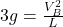## A uniform, thin rod of length L and mass M is allowed to pivot about its end. The rotational inertia of a rod about its end is ML2/3. The ro

Question

A uniform, thin rod of length L and mass M is allowed to pivot about its end. The rotational inertia of a rod about its end is ML2/3. The rod is fixed at one end and allowed to fall from the horizontal position through the vertical position. The student has a variety of rods of various lengths that all have a uniform mass distribution. Design an experiment that the student could conduct to find an experimental value of g.

in progress 0
6 months 2021-07-17T15:41:47+00:00 1 Answers 21 views 0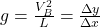Explanation:

Given that :

length of the thin rod = L

mass = m

The rotational inertia I =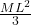The experimental design that the student can use to conduct the experimental value of g can be determined as follow:

Taking the integral value of I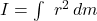where :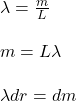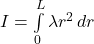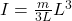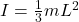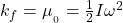where: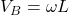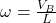Equating: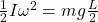; we have: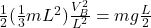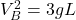since m = 3g

where :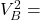vertical axis on the graph

L = horizontal axis( y = mx)